Magnetostatics MCQ Level - 1

# Magnetostatics MCQ Level - 1

Test Description

## 10 Questions MCQ Test Topic wise Tests for IIT JAM Physics | Magnetostatics MCQ Level - 1

Magnetostatics MCQ Level - 1 for IIT JAM 2022 is part of Topic wise Tests for IIT JAM Physics preparation. The Magnetostatics MCQ Level - 1 questions and answers have been prepared according to the IIT JAM exam syllabus.The Magnetostatics MCQ Level - 1 MCQs are made for IIT JAM 2022 Exam. Find important definitions, questions, notes, meanings, examples, exercises, MCQs and online tests for Magnetostatics MCQ Level - 1 below.
Solutions of Magnetostatics MCQ Level - 1 questions in English are available as part of our Topic wise Tests for IIT JAM Physics for IIT JAM & Magnetostatics MCQ Level - 1 solutions in Hindi for Topic wise Tests for IIT JAM Physics course. Download more important topics, notes, lectures and mock test series for IIT JAM Exam by signing up for free. Attempt Magnetostatics MCQ Level - 1 | 10 questions in 30 minutes | Mock test for IIT JAM preparation | Free important questions MCQ to study Topic wise Tests for IIT JAM Physics for IIT JAM Exam | Download free PDF with solutions
 1 Crore+ students have signed up on EduRev. Have you?
Magnetostatics MCQ Level - 1 - Question 1

### An electric current passes through a long straight wire. At a distance 5 cm from the wire, the magnetic field is B. The field at 20 cm from the wire would be

Detailed Solution for Magnetostatics MCQ Level - 1 - Question 1

The correct answer is: B/4

Magnetostatics MCQ Level - 1 - Question 2

### A magnetic field of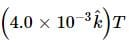exerts a force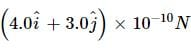on a particle having a charge 10−9C and moving in te x−y plane. Find the velocity of the particle.

Detailed Solution for Magnetostatics MCQ Level - 1 - Question 2

Given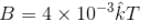q = 10–9 C
Magnetic force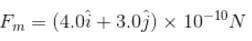Let velocity of the particle in x-y plane be,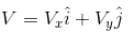Then from the relation
Fm =  q(V × B)
We have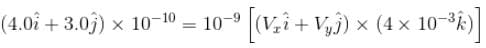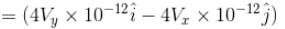Comparing the coefficient of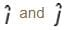we have,
4 × 10–10 = 4Vy × 10–12
Vy = 102 m/s = 100 m/s
and  3.0 × 10–10 = –4Vx × 10–12
Vx = –75 m/s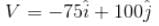The correct answer is: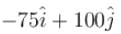Magnetostatics MCQ Level - 1 - Question 3

### The dimensional formula of magnetic induction is :

Detailed Solution for Magnetostatics MCQ Level - 1 - Question 3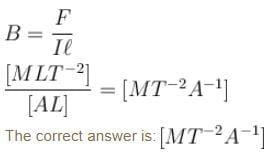Magnetostatics MCQ Level - 1 - Question 4

If a charge particle is describing a circle of radius r  in a magnetic field with a time period T. Then :

Detailed Solution for Magnetostatics MCQ Level - 1 - Question 4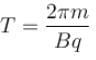So, T  is independent of  r.
The correct answer is: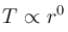Magnetostatics MCQ Level - 1 - Question 5

Two wires of same length are shaped into a square and a circle. If they carry same current, ratio of the magnetic moment is

Detailed Solution for Magnetostatics MCQ Level - 1 - Question 5

Let length of each wire is  L
For square length of each side  L/4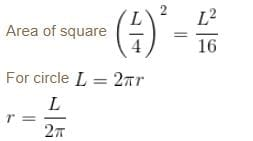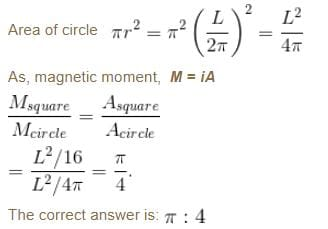Magnetostatics MCQ Level - 1 - Question 6

A positively charged particle falling freely under gravity enter a region having a uniform horizontal magnetic field pointing north to south the particle will be deflected towards

Detailed Solution for Magnetostatics MCQ Level - 1 - Question 6

Given that , V is pointing downwards and B is towards North

As magnetic force in given by,

f​m=q(V×B)

Using right hand thumb for negative charge we het the F is towards west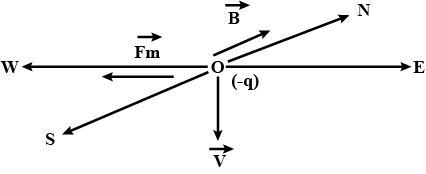Magnetostatics MCQ Level - 1 - Question 7

A charged particle enters a magnetic field H with its initial velocity making an angle of 45° with H the path of the particle will be :

Detailed Solution for Magnetostatics MCQ Level - 1 - Question 7

The correct answer is: a helix

Magnetostatics MCQ Level - 1 - Question 8

A wire carrying current i and other carrying current 2i  in the same direction produce a magnetic field B at the mid-point. What will be the field when 2i current is switched off?

Detailed Solution for Magnetostatics MCQ Level - 1 - Question 8

Magnetic field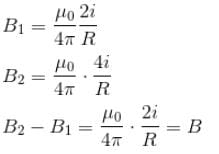B2 = 2B1
2B1 – B1 = B
B1 = B

Magnetostatics MCQ Level - 1 - Question 9

A long metallic cylinder of radius R has a current i flowing through it. The magnetic induction B  will vary with distance from the curve as shown in figure.

Detailed Solution for Magnetostatics MCQ Level - 1 - Question 9

The correct answer is: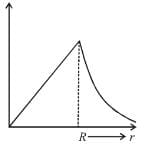Magnetostatics MCQ Level - 1 - Question 10

A proton and a deutron both having the same kinetic energy, enter perpendicularly into a uniform  magnetic field B. For motion of proton and deutron on circular path of radius Rp and Rd  respectively, the correct statement is :

Detailed Solution for Magnetostatics MCQ Level - 1 - Question 10

For charged particle on circular path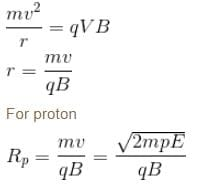For deutron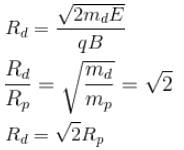The correct answer is: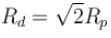## Topic wise Tests for IIT JAM Physics

217 tests
 Use Code STAYHOME200 and get INR 200 additional OFF Use Coupon Code
Information about Magnetostatics MCQ Level - 1 Page
In this test you can find the Exam questions for Magnetostatics MCQ Level - 1 solved & explained in the simplest way possible. Besides giving Questions and answers for Magnetostatics MCQ Level - 1, EduRev gives you an ample number of Online tests for practice

217 tests